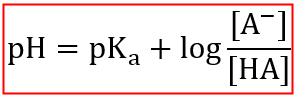# Problem: How much more acetic acid (pKa = 4.76) than acetate should be in solution to maintain a pH of 4.00?a. 2.13 times moreb. 5.75 times morec. 0.47 times mored. 0.00 times moree. 0.17 times more

###### FREE Expert Solution

Since the solution is a buffer, we can use the Henderson-Hasselbalch Equation to calculate the ratio of [CH3COO-]/[CH3COOH] to have a pH of 4.00:In the solution, identify the weak acid and the conjugate base. Recall from the Bronsted-Lowry definition that an acid is a proton (H+) donor and the base is a proton (H+) acceptor.

97% (200 ratings)###### Problem Details

How much more acetic acid (pKa = 4.76) than acetate should be in solution to maintain a pH of 4.00?

a. 2.13 times more

b. 5.75 times more

c. 0.47 times more

d. 0.00 times more

e. 0.17 times more